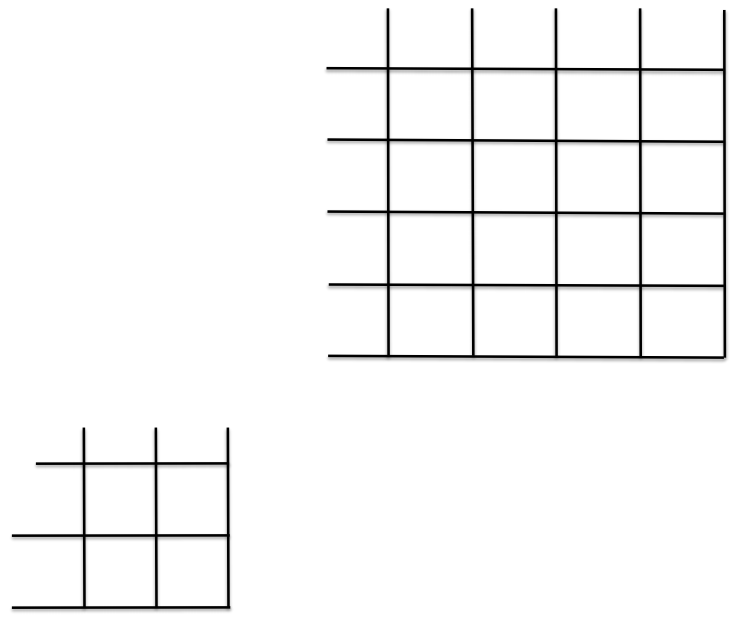Clutch Prep is now a part of Pearson
Ch.2 Mendel's Laws of InheritanceWorksheetSee all chapters

# Dihybrid Cross

See all sections
Sections
Mendel's Experiments and Laws
Inheritance in Diploids and Haploids
Monohybrid Cross
Dihybrid Cross
Probability and Genetics
Pedigrees

Concept #1: Punnet Square

Concept #2: Branch Diagram

Practice: Assume you have mated a homozygous dominant purple, square plant with a homozygous recessive pink, spherical plant. What is the proportion of purple and spherical plants that would be produced in the F2 generation?Practice: Write out all of the following gametes that can be produced from individuals with the following genotypes.

a. AaBB

b. AaBb

c. AaBbCc

d. AaBbcc

Practice: Two organisms with the genotypes Aa bb Cc Dd Ee and Aa Bb Cc dd Ee were crossed. Use the branch method to determine the proportion of the following genotypes in the offspring. I. aa bb cc dd ee

Practice: Two organisms with the genotypes Aa bb Cc Dd Ee and Aa Bb Cc dd Ee were crossed. Use the branch method to determine the proportion of the following genotypes in the offspring. II. Aa bb Cc dd ee

Practice: Two organisms with the genotypes Aa bb Cc Dd Ee and Aa Bb Cc dd Ee were crossed. Use the branch method to determine the proportion of the following genotypes in the offspring. III. AA BB CC Dd ee

Practice: In melons, spots (S) are dominant to no spots (s) and bitterness (B) is dominant to sweet (b). Answer the following questions that arise from a crossing of a homozygous dominant plant with a homozygous recessive plant. Assume Mendelian inheritance. I. What is the F2 phenotypic ratio if the F1 generation is intercrossed?